# The simplest non-Abelian example

It is worth considering the simplest non-abelian example more closely. The integer Heisenberg group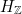is the simplest non-trivial example of a nilpotent group. It is generated by two elements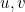with the relations that the commutator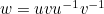commutes with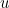and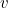. Explicitly, it is the group of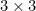upper-triangular matrices with integer entries and diagonal entries 1: takeandto be the matrices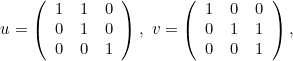so that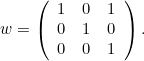Any element ofcan be written uniquely in the form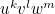for some integers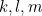.

The groupsits inside the more usual 3-dimensional Heisenberg group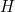consisting of the strictly upper-triangular matrices with real entries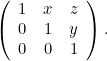By analogy with the case of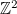in the plane, you might expect the groupto converge tounder re-scaling. As a manifold, the groupis just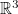, so you would predict thathas cubic polynomial growth just like the abelian group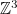. But actually it has quartic growth. This is easy to see: because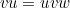we have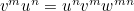for any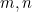, and so any of the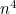elements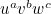with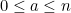,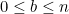, and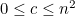can be obtained as the product of a string of at most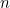copies ofand at mostcopies ofarranged in a suitable order. Why do we get quartic growth from such a 3-dimensional group? The answer takes us to a fascinating piece of geometry.

Althoughis identified with, we find that if we want left-multiplications in the group to be isometries we must warp the usual metric ofsomewhat. In fact the length of a path must be defined as the integral of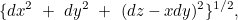which means that each plane perpendicular to the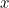-axis has been sheared in the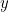-direction by an amount proportional to. As we re-scale the word-metric onwe must re-scale the metric ontoo, multiplyingandby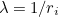, and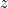by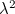. In the limit, this means that the only paths of finite length are those whose direction at each point lies in the plane given by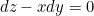. Let us call them the allowable paths. They give us a new metric onin which the distance from one point to another is the length of the shortest allowable path joining them. This metric defines the usual topology on, but, unlike the taxi-cab metric, it is far from equivalent — even locally — to the usual metric. Nevertheless, it is a metric which those of us who have tried to park a car know all too well. To move a car just a little bit sideways we must take it along a disproportionately long path. This is because the position of a car sitting on an expanse of tarmac is described by three coordinates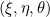, where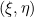is the position of the mid-point of the front wheels, and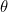is the angle in which the axis of symmetry of the car is pointing. When we want to move the car we can move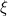and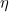any way we like, within reason, but the change in the third coordinate is constrained by the differential relation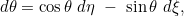assuming the car is of unit length. (A simple change of coordinates puts this in the form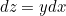which we found for the group.)

What is remarkable about this new metric is that on one hand it defines the usual topology of, but on the other hand it defines a metric space of Hausdorff dimension four. Hausdorff dimension is a concept defined only for metric spaces. To say it is four means, essentially, that the number of balls of radius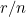needed to cover a ball of radius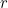grows likeas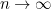. In the new metric onthis is the case because a small-ball for the new metric looks (in the usual coordinates) like a very flat ellipsoid with axes. This explains the quartic growth rate of the group. More generally, for any metric space the topological dimension is bounded above by the Hausdorff dimension, and so the finite-dimensionality of the limit space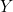follows from the polynomial growth of the group.

Back to the main article

• Want facts and want them fast? Our Maths in a minute series explores key mathematical concepts in just a few words.